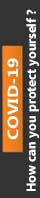We're Open

0% Plagiarism Guaranteed & Custom Written

# Find analytically the extrema of functions of two or more variables, with and without constraints,

Faculty of Science, Engineering and Computing

MA6000 Reassessment to replace in-class test

 Module: MA6000 Setters: Dr. Peter Soan Title of Assignment: Coursework Assignment 2 (Seen Test) Reassessment Deadline: 14/08/2020 Module weighting 20%

## ID Number:

### Max mark (points)

Q1 (Stationary Points)

25

Q2 (Golden Section Search)

9

Q3 (Hooke and Jeeves)

25

16

### Things to work on for next time

1.         Let . Find all of the stationary points of this function and, where possible, determine the nature of each stationary point.

( 20 marks)

If the function is slightly changed so that  where is a constant, one of the stationary points is . Give the range of  for which this stationary point is a maximum point.

( 5 marks)

2.  (a)     The Golden Section search method is to be applied to a unimodal function to find the minimum in the interval [3, 15].  If the interval of uncertainty around the calculated optimum point is required to be not longer than 0.03, determine how many iterations are needed for the Golden Section search? (You may assume that the ratio for symmetric interval subdivision is rk= r =0.382.).

(2 marks)

(b)     Use the Golden Section search method to find the minimum of  in the interval [0, 1.8] if the interval of uncertainty around the calculated optimum point (x value) is required to be not longer than 0.2. Work to 4 decimal places accuracy.

.           (7 marks)

(At the ith iteration of a Golden Section search the new interval of uncertainty uses the calculation of  or  where ,  and  is the current interval of uncertainty.)

3.         Hooke and Jeeves’ method may be used to solve constrained optimisation problems by the simple device of regarding as a failure any exploratory or pattern move which takes the current point out of the feasible region. (Work through the Hooke and Jeeves algorithm exactly as normal, but within that process classify any point outside the feasible region as a failure regardless of whether or not the function value is lower than previous values.) Use this technique to minimise

subject to.

(Reject any point for which the above constraint is not satisfied.)

Take  as the initial point,  as initial step lengths and stop when . Do not perform more than 5 pattern moves.

(25 marks)

4.         Consider the following function of two variables.

(i)         Find the stationary points of this function and attempt to classify them using the second order (Hessian) condition.  If this test is not appropriate state why you would expect the stationary points to be of a particular type.

(4 marks)

(ii)        Find the local minimum of the above function using Nelder and Mead’s method.  Start from the simplex defined by (1,2), (2,2) and (1,3)  and terminate the procedure either after four iterations or when you need to reduce the size of the simplex by halving the distances from .

(12 marks)

You may assume the following for Nelder and Mead’s method:

If, and denote the vertices with the highest, the lowest and the second highest function value respectively, then the centroid  is given by:

Reflection:

Expansion ():

1

If  keep  otherwise

Contraction ():

If   then

If   then

If  and   keep  otherwise reduce.

End of Coursework Assignment

100% Plagiarism Free & Custom Written,International House, 12 Constance Street, London, United Kingdom,
E16 2DQ

## STILL NOT CONVINCED?

We've produced some samples of what you can expect from our Academic Writing Service - these are created by our writers to show you the kind of high-quality work you'll receive. Take a look for yourself!FLAT 25% OFF ON EVERY ORDER.Use "FLAT25" as your promo code during checkout